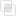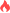Python列表操作方法的整理

python列表2021-06-01 17:07:07511浏览 · 0收藏 · 0评论1、append用于在列表末尾追加新的对象

```a = [1,2,3]
a.append(4)                          #the result ： [1, 2, 3, 4]```

2、count方法统计某个元素在列表中出现的次数

```a = ['aa','bb','cc','aa','aa']
print(a.count('aa'))                 #the result ： 3```

3、extend方法可以在列表的末尾一次性追加另一个序列中的多个值

```a = [1,2,3]
b = [4,5,6]
a.extend(b)                          #the result ：[1, 2, 3, 4, 5, 6]```

4、 index函数用于从列表中找出某个值第一个匹配项的索引位置

```a = [1,2,3,1]
print(a.index(1))                   #the result ： 0```

5、insert方法用于将对象插入到列表中

```a = [1,2,3]
a.insert(0,'aa')            #the result : ['aa', 1, 2, 3]```

6、pop方法会移除列表中的一个元素（默认是最后一个），并且返回该元素的值

```a = [1,2,3]
a.pop()                             #the result ： [1, 2]
a.pop(0)```python列表如何分片操作？python列表中删除元素的三种方法python列表运算详解Python列表常见操作总结Python列表两种排序类型的实现python列表的优点探究python列表生成式的两种语法python列表(list)和元组(tuple)详解python列表读取的方法python列表如何追加元素python列表中有哪些内置函数python列表追加元素出错的解决python列表排序的两种方式python列表的数据类型分析python列表如何结合数组使用python列表的多行写法python列表索引的两种用法python列表如何传递到线程？python列表的创建和存放python列表添加和删除的方法python列表操作符有哪些python列表中sort()参数的使用Python列表中有哪些索引python异常中else的使用890

python捕获多个异常的规则1181

python ReLU函数是什么1235